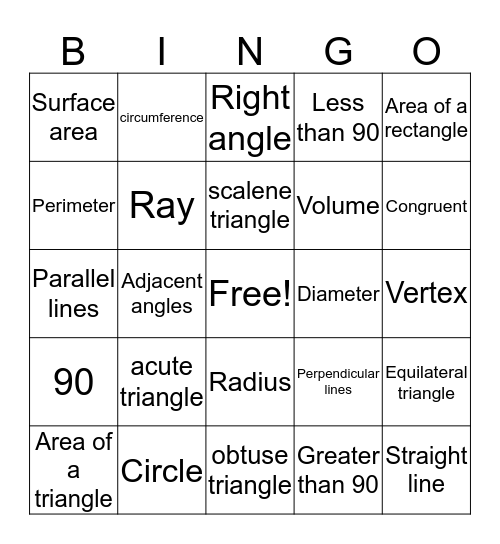# Geometry BingoThis bingo card has a free space and 25 words: acute triangle, obtuse triangle, scalene triangle, Area of a triangle, Area of a rectangle, Surface area, Perimeter, circumference, Diameter, Radius, Volume, Parallel lines, Perpendicular lines, Equilateral triangle, Ray, Line segment, Vertex, Right angle, Congruent, Adjacent angles, Straight line, Circle, 90, Less than 90 and Greater than 90.

## Play Online